## 10-year anniversary

### Featured

July 15th, 2022

I started writing this blog 10 years ago and it is to date one of the things I am the most proud of.

This is a big milestone for me, so I would like to begin with thanking all the people that encouraged me at the beginning, and in particular, for their valiant support and feedback: Matt Hall, Evan Bianco, Oliver Kuhn, Steve Lynch, and last but not least my life partner Rebecca.

A lot of the work I did in the first couple of years was on furthering my understanding, and sharing that with others, of the use of colours in scientific visualization, and how to use better colormaps, for example Perceptual rainbow palette, part a and part b.

I am grateful I achieved those knowledge-sharing goals:

• The material is referenced in the matplotlib documentation
• The blog has often been used as reference in talks and other publications on colormaps, beginning with this classic matplotlib talk given by Kristen Thyng at scipy 2014
• I was thrilled to have received positive feedback on my work by Bernice Rogovitz, someone I hold in very high esteem
• Some of that work on the blog resulted in being invited by Matt Hall to write a tutorial for The Leading Edge (SEG). The tutorial came with a Jupyter notebook demonstrating how to evaluate default colour maps and favour more perceptual alternatives
• I am particularly proud to see that the article is still ranking in the top 20 most downloaded papers from The Leading Edge (between 2010-2020)
• Additionally, the two blog post are to date top results for the #google search “perceptual rainbow” and “perceptual palette images

Ultimately, I am very happy to have created a space for sharing and exchanging ideas freely.

So, to celebrate these 10 years of MyCarta, I treated it to a new domain, mycartablog.com (but the old domain, and links still work) and a brand new look (it took me a while to get there but I like it a lot) with a theme that should now be responsive for all devices (welcome to the new era Matteo!).

I will also soon publish a short series of short but sweet new posts on colormaps and visualization (and republish on linkedin).

Thank you all for sharing this journey!

# Geoscience Machine Learning bits and bobs – data completeness

#### 2016 Machine learning contest – Society of Exploration Geophysicists

In a previous post I showed how to use `pandas.isnull` to find out, for each well individually, if a column has any null values, and `sum` to get how many, for each column. Here is one of the examples (with more modern, pandaish syntax compared to the example in the previous post:

```for well, data in training_data.groupby('Well Name'):
print(well)
print (data.isnull().values.any())
print (data.isnull().sum(), '\n')
```

Simple and quick, the output showed met that  – for example – the well ALEXANDER D is missing 466 samples from the PE log:

```ALEXANDER D
True
Facies         0
Formation      0
Well Name      0
Depth          0
GR             0
ILD_log10      0
DeltaPHI       0
PHIND          0
PE           466
NM_M           0
RELPOS         0
dtype: int64```

A more appealing and versatile alternative, which I discovered after the contest, comes with the `matrix` function form the missingno library. With the code below I can turn each well into a Pandas DataFrame on the fly, then a missingno matrix plot.

```for well, data in training_data.groupby('Well Name'):

msno.matrix(data, color=(0., 0., 0.45))
fig = plt.gcf()
fig.set_size_inches(20, np.round(len(data)/100)) # heigth of the plot for each well reflects well length
axes=fig.get_axes()
axes.set_title(well, color=(0., 0.8, 0.), fontsize=14, ha='center')```

I find that looking at these two plots provides a very compelling and informative way to inspect data completeness, and I am wondering if they couldn’t be used to guide the strategy to deal with missing data, together with domain knowledge from petrophysics.

Interpreting the dendrogram in a top-down fashion, as suggested in the library documentation, my first thoughts are that this may suggest trying to predict missing values in a sequential fashion rather than for all logs at once. For example, looking at the largest cluster on the left, and starting from top right, I am thinking of testing use of GR to first predict missing values in RDEP, then both to predict missing values in RMED, then DTC. Then add CALI and use all logs completed so far to predict RHOB, and so on.

Naturally, this strategy will need to be tested against alternative strategies using lithology prediction accuracy. I would do that in the context of learning curves: I am imagining comparing the training and crossvalidation error first using only non NaN rows, then replace all NANs with mean, then compare separately this sequential log completing strategy with an all-in one strategy.

# Busting bad colormaps with Python and Panel

I have not done much work with, or written here on the blog about colormaps and perception in quite some time.

Last spring, however, I decided to build a web-based app to show the effects of using a bad colormaps. This stemmed from two needs: first, to further my understanding of Panel, after working through the awesome tutorial by James Bednar, Panel: Dashboards (at PyData Austin 2019); and second, to enable people to explore interactively the effects of bad colormaps on their perception, and consequently the ability to on interpret faults on a 3D seismic horizon.

I introduced the app at the Transform 2020 virtual subsurface conference, organized by Software Underground last June. Please watch the recording of my lightning talk as it explains in detail the machinery behind it.

I am writing this post in part to discuss some changes to the app. Here’s how it looks right now: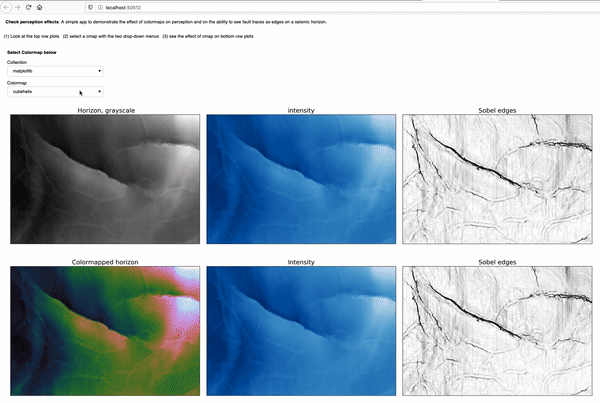The most notable change is the switch from one drop-down selector to two-drop-down selectors, in order to support both the Matplotlib collection and the Colorcet collection of colormaps. Additionally, the app has since been featured in the resource list on the Awesome Panel site, an achievement I am really proud of.You can try the app yourself by either running the notebook interactively with Binder, by clicking on the button below:```https://mybinder.org/v2/gh/mycarta/Colormap-distorsions-Panel-app/master?urlpath=%2Fpanel%2FDemonstrate_colormap_distortions_interactive_Panel
```

Let’s look at a couple of examples of insights I gained from using the app. For those that jumped straight to this example, the top row shows:

• the horizon, plotted using the benchmark grayscale colormap, on the left
• the horizon intensity, derived using `skimage.color.rgb2gray`, in the middle
• the Sobel edges detected on the intensity, on the right

and the bottom row,  shows:

• the horizon, plotted using the Matplotlib `gist_rainbow` colormap, on the left
• the intensity of the colormapped, in the middle. This is possible thanks to a function that makes a figure (but does not display it), plots the horizon with the specified colormap, then saves plot in the canvas to an RGB numpy array
• the Sobel edges detected on the colormapped intensity, on the rightI think the effects of this colormaps are already apparent when comparing the bottom left plot to the top left plot. However, simulating perception can be quite revealing for those that have not considered these effects before. The intensity in the bottom middle plot is very washed out in the areas corresponding to green color in the bottom left, and as a result, many of the faults are not visible any more, or only with much difficulty, which is demonstrated by the Sobel edges in the bottom right.

And if you are not quite convinced yet, I have created these hill-shaded maps, using Matt Hall”s delightful function from this notebook (and check his blog post):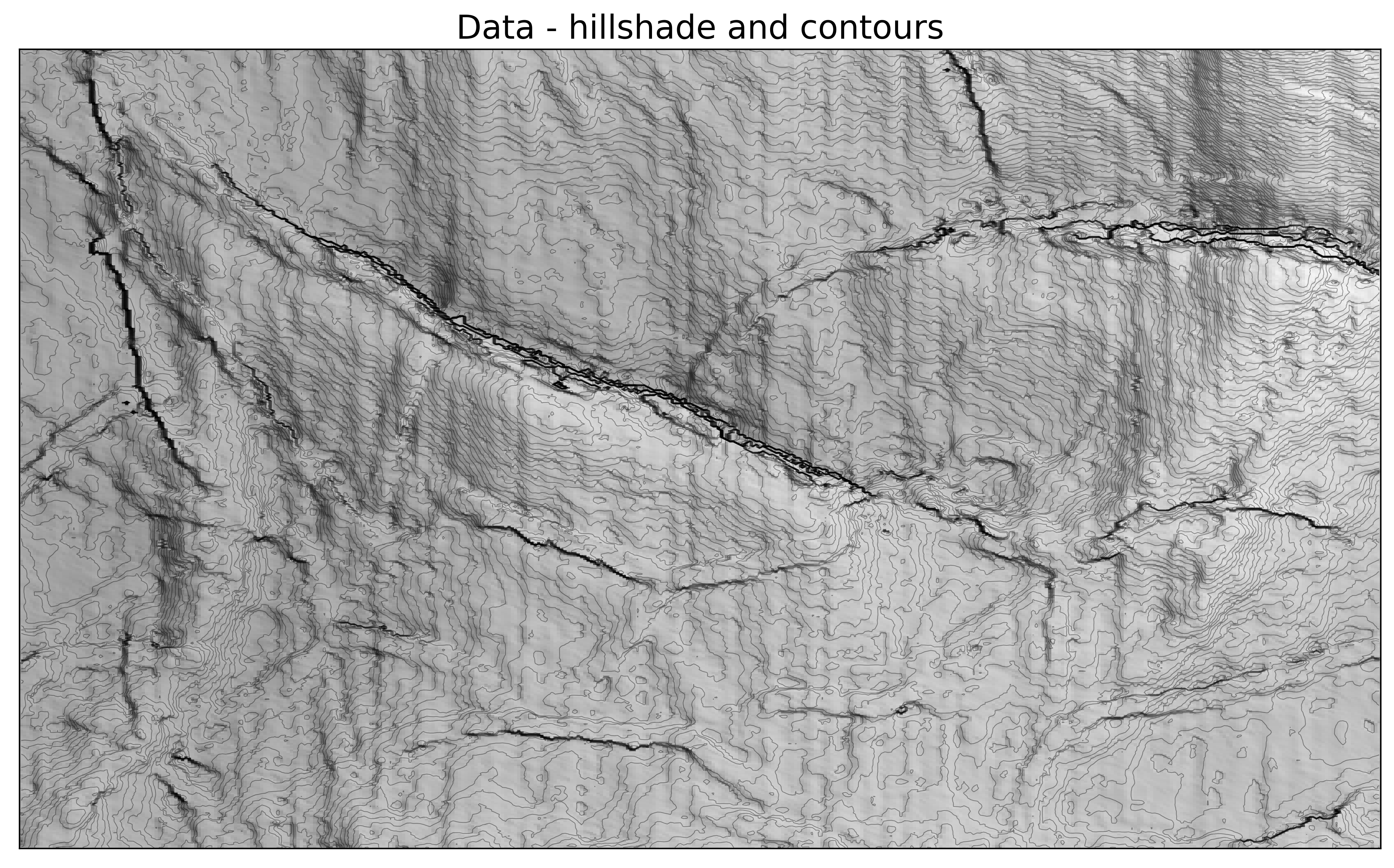Below is another example, using the Colocrcet `cet_rainbow` which is is one of Peter Kovesi’s perceptually uniform colormaps.  I use many of Peter’s colormaps, but never used this one, because I use my own perceptual rainbow, which does not have  a fully saturated yellow, or a fully saturated red. I think the app demonstrate, that even though they are more subtle , this rainbow still is introducing some artifacts. The yellow colour creates narrow flat bands, visible in the intensity and Sobel plots, and indicated by yellow arrows; the red colour is also bad as usual, causing an artificial decrease in intensity(magenta arrows).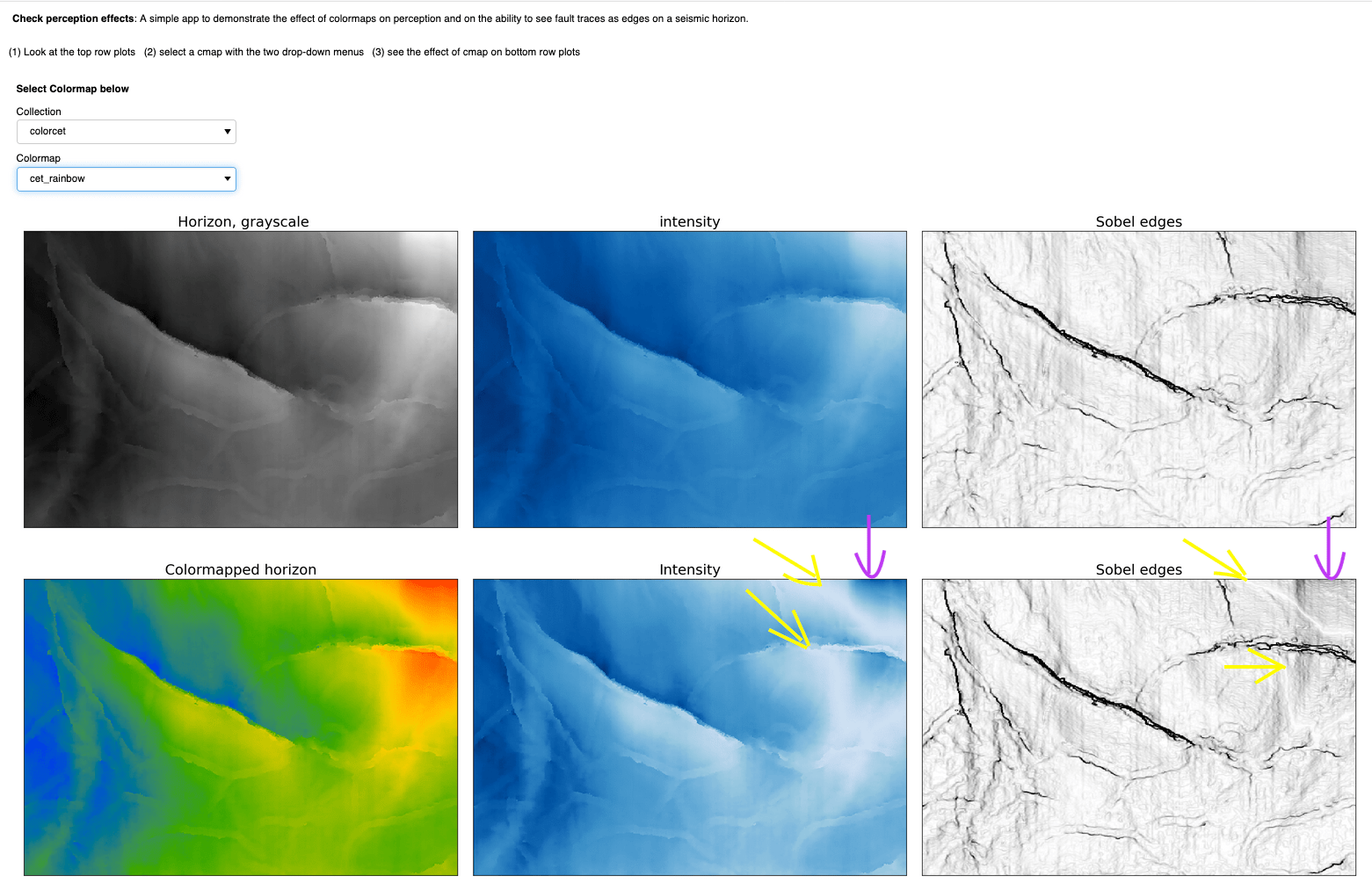## Data exploration in Python: distance correlation and variable clustering

### Featured

April 10, 2019

In my last post I wrote about visual data exploration with a focus on correlation, confidence, and spuriousness. As a reminder to aficionados, but mostly for new readers’ benefit: I am using a very small toy dataset (only 21 observations) from the paper Many correlation coefficients, null hypotheses, and high value (Hunt, 2013).

The dependent/target variable is oil production (measured in tens of barrels of oil per day) from a marine barrier sand. The independent variables are: Gross pay, in meters; Phi-h, porosity multiplied by thickness, with a 3% porosity cut-off; Position within the reservoir (a ranked variable, with 1.0 representing the uppermost geological facies, 2.0 the middle one, 3.0 the lowest one); Pressure draw-down in MPa. Three additional ‘special’ variables are: Random 1 and Random 2, which are range bound and random, and were included in the paper, and Gross pay transform, which I created specifically for this exercise to be highly correlated to Gross pay, by passing Gross pay to a logarithmic function, and then adding a bit of normally distributed random noise.

##### Correlation matrix with ellipses

I am very pleased with having been able to put together, by the end of it, a good looking scatter matrix that incorporated:

• bivariate scatter-plots in the upper triangle, annotated with rank correlation coefficient, confidence interval, and probability of spurious correlation
• contours in the lower triangle
• shape of the bivariate distributions (KDE) on the diagonal

In a comment to the post, Matt Hall got me thinking about other ways to visualize the correlation coefficient.  I did not end up using a colourmap for the facecolour of the plot (although this would probably be relatively easy, in an earlier attempt using hex-bin plots, the colourmap scaling of each plot independently – to account for outliers – proved challenging). But after some digging I found the Biokit library, which comes with a lot of useful visualizations, among which `corrplot` is exactly what I was looking for. With only a bit of tinkering I was able to produce, shown in Figure 1, a correlation matrix with:

• correlation coefficient in upper triangle (colour and intensity indicate whether positive or negative correlation, and its strength, respectively)
• bivariate ellipses in the lower triangle (ellipse direction and colour indicates whether positive or negative correlation; ellipticity and colour intensity are proportional to the correlation coefficient)Figure 1. Correlation matrix using the Biokit library

Also notice that – quite conveniently – the correlation matrix of Figure 1 is reordered with strongly correlated variables adjacent to one another, which facilitates interpretation. This is done using the rank correlation coefficient, with `pandas.DataFrame.corr`, and Biokit’s `corrplot`:

```corr = data.corr(method='spearman')
c = corrplot.Corrplot(corr)
c.plot(method='ellipse', cmap='PRGn_r', shrink=1, rotation=45, upper='text', lower='ellipse')
fig = plt.gcf()
fig.set_size_inches(10, 8);```

The insightful take-away is that with this reordering, the more ‘interesting’ variables, because of strong correlation (as defined in this case by the rank correlation coefficient), are close together and reposition along the diagonal, so we can immediately appreciate that Production, Phi-h, and Gross Pay, plus to a lesser extent position (albeit this one with negative correlation to production) are related to one another. This is a great intuition, and supports up our hypothesis (in an inferential test), backed by physical expectation, that production should be related to those other quantities.

But I think it is time to move away from either Pearson or Spearman correlation coefficient.

##### Correlation matrix with distance correlation and its p-value

I learned about distance correlation from Thomas when we were starting to work on our 2018 CSEG/CASP Geoconvention talk Data science tools for petroleum exploration and production“. What I immediately liked about distance correlation is that it does not assume a linear relationship between variables, and even more importantly, whereas with Pearson and Spearman a correlation value of zero does not prove independence between any two variables, a distance correlation of zero does mean that there is no dependence between those two variables.

Working on the R side, Thomas used the Energy inferential statistic package. With the`dcor` function he calculated the distance correlation, and with the `dcov.test `function the p-value via bootstrapping.

For Python, I used the `dcor` and `dcor.independence.distance_covariance_test` from the dcor library (with many thanks to Carlos Ramos Carreño, author of the Python library, who was kind enough to point me to the table of energy-dcor equivalents). So, for example, for one variable pair, we can do this:

```print ("distance correlation = {:.2f}".format(dcor.distance_correlation(data['Production'],
data['Gross pay'])))
print("p-value = {:.7f}".format(dcor.independence.distance_covariance_test(data['Production'],
data['Gross pay'],
exponent=1.0,
num_resamples=2000)))
>>> distance correlation  = 0.91
>>> p-value = 0.0004998```

So, wanting to apply these tests in a pairwise fashion to all variables, I modified the `dist_corr` function and `corrfunc` function from the existing notebook

```def dist_corr(X, Y, pval=True, nruns=2000):
""" Distance correlation with p-value from bootstrapping
"""
dc = dcor.distance_correlation(X, Y)
pv = dcor.independence.distance_covariance_test(X, Y, exponent=1.0, num_resamples=nruns)
if pval:
return (dc, pv)
else:
return dc```
```def corrfunc(x, y, **kws):
d, p = dist_corr(x,y)
#print("{:.4f}".format(d), "{:.4f}".format(p))
if p > 0.1:
pclr = 'Darkgray'
else:
pclr= 'Darkblue'
ax = plt.gca()
ax.annotate("DC = {:.2f}".format(d), xy=(.1, 0.99), xycoords=ax.transAxes, color = pclr, fontsize = 14)```

so that I can then just do:

```g = sns.PairGrid(data, diag_sharey=False)
axes = g.axes
g.map_upper(plt.scatter, linewidths=1, edgecolor="w", s=90, alpha = 0.5)
g.map_upper(corrfunc)
g.map_diag(sns.kdeplot, lw = 4, legend=False)
g.map_lower(sns.kdeplot, cmap="Blues_d")
plt.show();```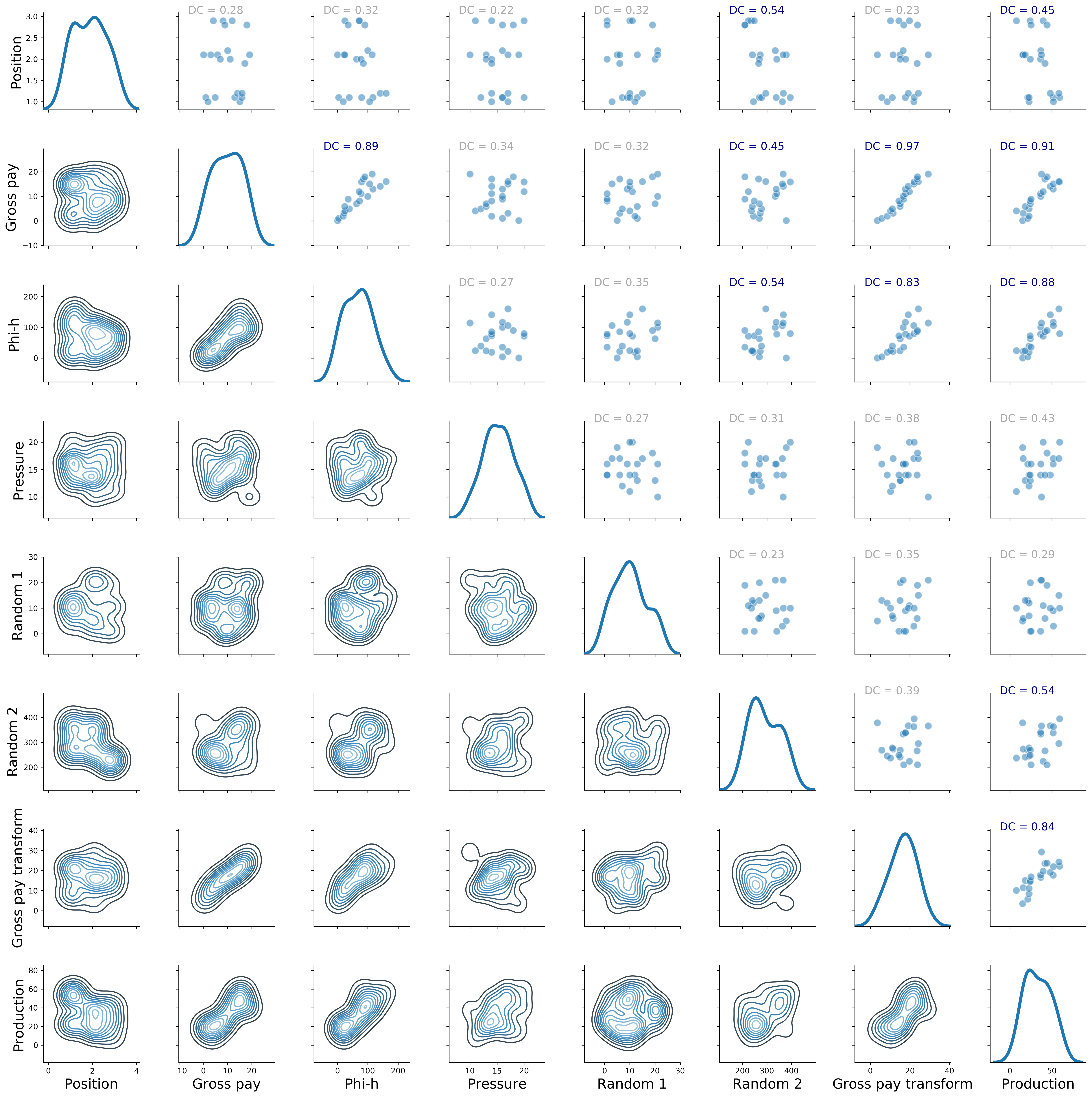Figure 2. Revised Seaborn pairgrid matrix with distance correlation colored by p-value (gray if p > 0.10, blue if p <= 0.10)

##### Clustering using distance correlation

I really like the result in Figure 2.  However, I want to have more control on how the pairwise plots are arranged; a bit like in Figure 1, but using my metric of choice, which would be again the distance correlation. To do that, I will first show how to create a square matrix of distance correlation values, then I will look at clustering of the variables; but rather than passing the raw data to the algorithm, I will pass the distance correlation matrix. Get ready for a ride!

For the first part, making the square matrix of distance correlation values, I adapted the code from this brilliant SO answer on Euclidean distance (I recommend you read the whole answer):

```# Create the distance method using distance_correlation
distcorr = lambda column1, column2: dcor.distance_correlation(column1, column2)
# Apply the distance method pairwise to every column
rslt = data.apply(lambda col1: data.apply(lambda col2: distcorr(col1, col2)))
# check output
pd.options.display.float_format = '{:,.2f}'.format
rslt
```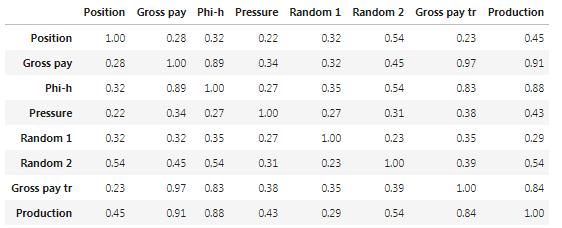Table I. Distance correlation matrix.

The matrix in Table I looks like what I wanted, but let’s calculate a couple of values directly, to be sure:

```print ("distance correlation = {:.2f}".format(dcor.distance_correlation(data['Production'],
data['Phi-h'])))
print ("distance correlation = {:.2f}".format(dcor.distance_correlation(data['Production'],
data['Position'])))```
```>>> distance correlation = 0.88
>>> distance correlation = 0.45```

Excellent, it checks!

Now I am going to take a bit of a detour, and use that matrix, rather than the raw data, to cluster the variables, and then display the result with a heat-map and accompanying dendrograms. That can be done with Biokit’s `heatmap`:

```data.rename(index=str, columns={"Gross pay transform": "Gross pay tr"}, inplace=True)
distcorr = lambda column1, column2: dcor.distance_correlation(column1, column2)
rslt = data.apply(lambda col1: data.apply(lambda col2: distcorr(col1, col2)))
h = heatmap.Heatmap(rslt)
h.plot(vmin=0.0, vmax=1.1, cmap='cubehelix')
fig = plt.gcf()
fig.set_size_inches(22, 18)
plt.gcf().get_axes().invert_xaxis();
```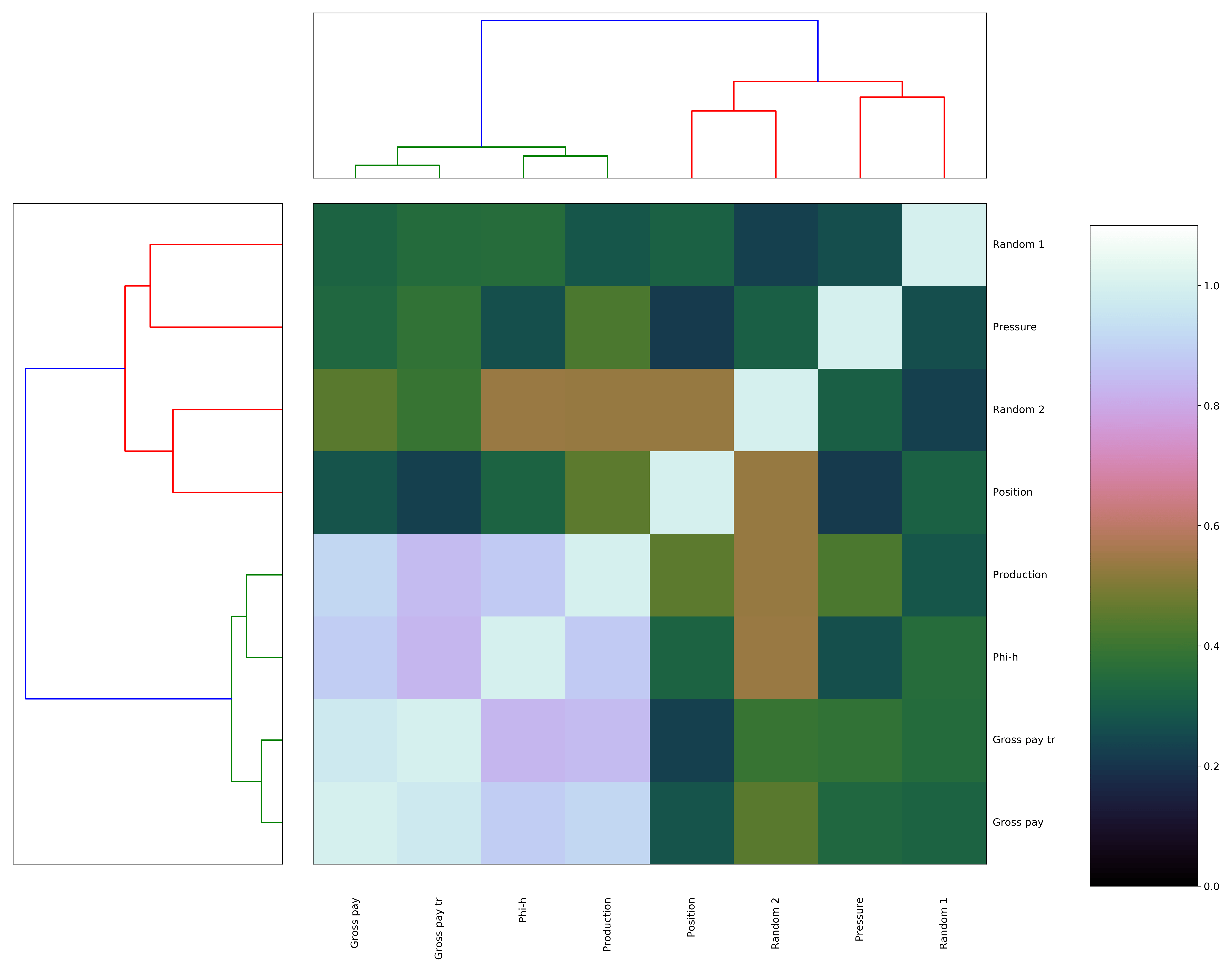Figure 3. Biokit heatmap with dendrograms, using correlation distance matrix

That’s very nice, but please notice how much ‘massaging’ it took: first, I had to flip the axis for the dendrogram for the rows (on the left) because it would be incorrectly reversed by default; and then, I had to shorten the name of Gross-pay transform so that its row label would not end up under the colorbar (also, the diagonal is flipped upside down, and I could not reverse it or else the colum labels would go under the top dendrogram). I suppose the latter too could be done on the Matplotlib side, but why bother when we can get it all done much more easily with Seaborn? Plus, you will see below that I actually have to… but I’m putting the cart before the horses…. here’s the Seaborn code:

```g = sns.clustermap(rslt, cmap="mako",  standard_scale =1)
fig = plt.gcf()
fig.set_size_inches(18, 18);```

and the result in Figure 4. We really get everything for free!Figure 4. Seaborn clustermap, using correlation distance matrix

Before moving to the final fireworks, a bit of interpretation: again what comes up is that Production, Phi-h, Gross Pay, and Gross pay transform group together, as in Figure 1, but now the observation is based on a much more robust metric. Position is not as ‘close’, it is ends up in a different cluster, although its distance correlation from Production is 0.45, and the p-value is <0.10, hence it is still a relevant variable.

I think this is as far as I would go with interpretation. It does also show me that Gross pay, and Gross pay transform are very much related to one another, with high dependence, but it does still not tell me, in the context of variable selection for predicting Production, which one I should drop: I only know it should be Gross pay transform because I created it myself. For proper variable selection I will look at techniques like Least Absolute Shrinkage and Selection Operator (LASSO, which Thomas has showcased in his R notebook) and Recursive Feature Elimination (I’ll be testing Sebastan Raschka‘s Sequential Feature Selector from the `mlxtend` library).

##### Correlation matrix with distance correlation, p-value, and plots rearranged by clustering

I started this whole dash by saying I wanted to control how the pairwise plots were arranged in the scatter matrix, and that to do so required use of  Seaborn. Indeed, it turns out the reordered row/column indices (in our case they are the same) can be easily accessed:

```a = (g.dendrogram_col.reordered_ind)
print(a)
>>> [1, 6, 2, 7, 0, 5, 3, 4]```

and if this is the order of variables in the original DataFrame:

```b = list(data)
print (b)
>>> [Position', 'Gross pay', 'Phi-h', 'Pressure', 'Random 1', 'Random 2', 'Gross pay transform', 'Production']```

we can rearrange them with those reordered column indices:

```data = data[[b[i] for i in a]]
print(list(data))
>>> ['Gross pay', 'Gross pay transform', 'Phi-h', 'Production', 'Position', 'Random 2', 'Pressure', 'Random 1']```

after which we can simply re-run the code below!!!

```g = sns.PairGrid(data, diag_sharey=False)
axes = g.axes
g.map_upper(plt.scatter, linewidths=1, edgecolor="w", s=90, alpha = 0.5)
g.map_upper(corrfunc)
g.map_diag(sns.kdeplot, lw = 4, legend=False)
g.map_lower(sns.kdeplot, cmap="Blues_d")
plt.show()
```Figure 5. Revised Seaborn pairgrid matrix with distance correlation colored by p-value (gray if p > 0.10, blue if p <= 0.10), and plots rearranged by clustering results

# Visual data exploration in Python – correlation, confidence, spuriousness

This weekend – it was long overdue –  I cleaned up a notebook prepared last year to accompany the talk “Data science tools for petroleum exploration and production“, which I gave with my friend Thomas Speidel at the Calgary 2018 CSEG/CSPG Geoconvention.

The Python notebook can be downloaded from GitHub as part of a full repository, which includes R code from Thomas, or run interactively with Binder.

In this post I want to highlight on one aspect in particular: doing data exploration visually, but also quantitatively with inferential statistic tests.

Like all data scientists (professional, or in the making, and I ‘park’ myself for now in the latter bin), a scatter matrix is often the first thing I produce, after data cleanup, to look for obvious pairwise relationships and trends between variables. And I really love the flexibility, and looks of `seaborn`‘s `pairgrid`.

In the example below I use again data from Lee Hunt, Many correlation coefficients, null hypoteses, and high value CSEG Recorder, December 2013; my followers would be familiar with this small toy dataset from reading Geoscience ML notebook 2 and Geoscience_ML_notebook 3.

The scatter matrix in Figure 1 includes bivariate scatter-plots in the upper triangle, contours in the lower triangle, shape of the bivariate distributions on the diagonal.

```matplotlib.pyplot.rcParams["axes.labelsize"] = 18
g = seaborn.PairGrid(data, diag_sharey=False)
axes = g.axes
g.map_upper(matplotlib.pyplot.scatter,  linewidths=1,
edgecolor="w", s=90, alpha = 0.5)
g.map_diag(seaborn.kdeplot, lw = 4, legend=False)
g.map_lower(seaborn.kdeplot, cmap="Blues_d")
matplotlib.pyplot.show()```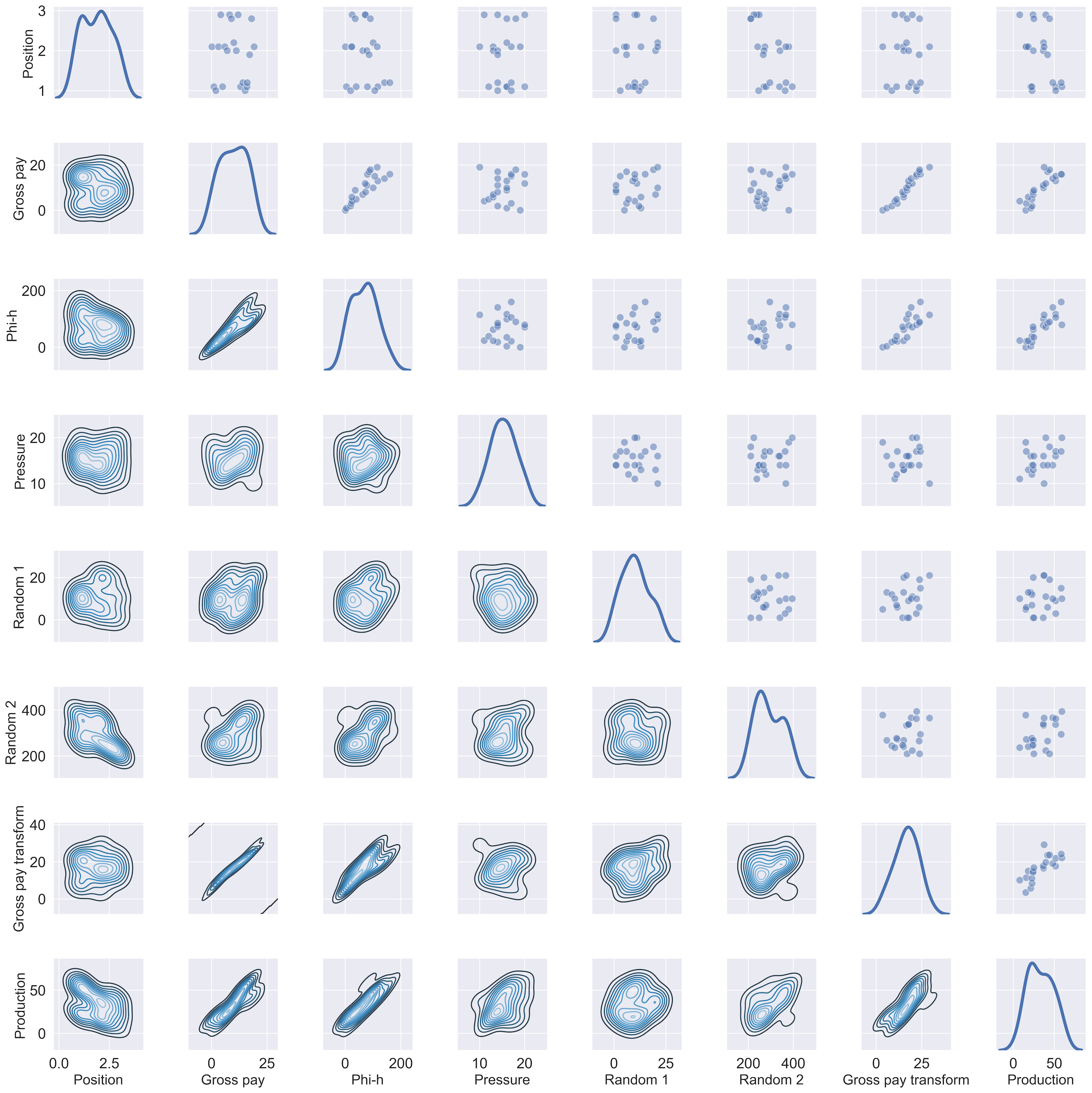Scatter matrix from pairgrid

It looks terrific. But, as I said, I’d like to show how to add to the individual scatterplots some useful annotation. These are the ones I typically focus on:

For the Spearman correlation coefficient I use `scipy.stats.spearmanr`, whereas for the confidence interval and the probability of spurious correlation I use my own functions, which I include below (following, respectively, Stan Brown’s  Stats without tears and Cynthia Kalkomey’s Potential risks when using seismic attributes as predictors of reservoir properties):

```def confInt(r, nwells):
z_crit = scipy.stats.norm.ppf(.975)
std_Z = 1/numpy.sqrt(nwells-3)
E = z_crit*std_Z
Z_star = 0.5*(numpy.log((1+r)/(1.0000000000001-r)))
ZCI_l = Z_star - E
ZCI_u = Z_star + E
RCI_l = (numpy.exp(2*ZCI_l)-1)/(numpy.exp(2*ZCI_l)+1)
RCI_u = (numpy.exp(2*ZCI_u)-1)/(numpy.exp(2*ZCI_u)+1)
return RCI_u, RCI_l```
```def P_spurious (r, nwells, nattributes):
t_of_r = r * numpy.sqrt((nwells-2)/(1-numpy.power(r,2)))
p = scipy.stats.t.sf(numpy.abs(t_of_r), nwells-2)*2
ks = numpy.arange(1, nattributes+1, 1)
return numpy.sum(p * numpy.power(1-p, ks-1))```

I also need a function to calculate the critical r, that is, the value of correlation coefficient  above which one can rule out chance as an explanation for a relationship:

```def r_crit(nwells, a):
t = scipy.stats.t.isf(a, nwells-2)
r_crit = t/numpy.sqrt((nwells-2)+ numpy.power(t,2))
return r_crit```

where `a`   is equal to `alpha/2`, alpha being the level of significance, or the chance of being wrong that one accepts to live with, and `nwells-2` is equivalent to the degrees of freedom.

Finally, I need a utility function (adapted from this Stack Overflow answer) to calculate on the fly and annotate the individual scatterplots with the CC, CI, and P.

```def corrfunc(x, y, rc=rc, **kws):
r, p = svipy.stats.spearmanr(x, y)
u, l = confInt(r, 21)
if r > rc:
rclr = 'g'
else:
rclr= 'm'
if p > 0.05:
pclr = 'm'
else:
pclr= 'g'
ax = matplotlib.pyplot.gca()
ax.annotate("CC = {:.2f}".format(r), xy=(.1, 1.25),
xycoords=ax.transAxes, color = rclr, fontsize = 14)
ax.annotate("CI = [{:.2f} {:.2f}]".format(u, l), xy=(.1, 1.1),
xycoords=ax.transAxes, color = rclr, fontsize = 14)
ax.annotate("PS = {:.3f}".format(p), xy=(.1, .95),
xycoords=ax.transAxes, color = pclr, fontsize = 14)```

So, now I just calculate the critical r for the number of observations, 21 wells in this case:

`rc = r_crit(21, 0.025)`

and wrap it all up this way:

```matplotlib.pyplot.rcParams["axes.labelsize"] = 18
g = seaborn.PairGrid(data, diag_sharey=False)
axes = g.axes
g.map_upper(matplotlib.pyplot.scatter,  linewidths=1,
edgecolor="w", s=90, alpha = 0.5)
g.map_upper(corrfunc)
g.map_diag(seaborn.kdeplot, lw = 4, legend=False)
g.map_lower(seaborn.kdeplot, cmap="Blues_d")
matplotlib.pyplot.show()```

so that:

• the correlation coefficient is coloured green if it is larger than the critical r, else coloured in purple
• the confidence interval is coloured green if both lower and upper are larger than the critical r, else coloured in purple
• the probability of spurious correlation is coloured in green when below 0.05 (or 5% chance)Enhanced scatter matrix

# Geophysics Python sprint 2018 – day 1

Last weekend I went to California to attend my first ever Python sprint, which was organized at MAZ Café con leche (Santa Ana) by Agile Scientific.

For me this event was a success in many respects. First of all, I wanted to spend some dedicated time working on an open source project, rather than chipping away at it once in a while. Also, participating in a project that was not my own seemed like a good way to challenge myself, by pushing me out of a zone of comfort. Finally, this was an opportunity to engage with other members of the Software Underground Slack team, some of which (for example Jesper Dramsch and Brendon Hall) I’ve known for some time but actually never met in person.

Please read about the Sprint in general on Matt Hall‘s blog post, Café con leche. My post is a short summary of what I did on the first day.

After a tasty breakfast, and at least a good hour of socializing, I sat at a table with three other people interested in working on Bruges (Agile’s Python library for Geophysics) : Jesper Dramsch, Adriana Gordon and Volodymyr Vragov.

As I tweeted that evening, we had a light-hearted start, but then we set to work.While Adriana and Jesper tackled Bruges’ documentation, which was sorely needed, Volodymyr spent some hours on example notebooks from in-Bruges (a tour of Bruges), which needed fixing, and also on setting up our joint project for day 2 (more in the next post). For my part, I  put together a tutorial notebooks on how to use Bruges’ functions on wireline logs stored in a Pandas DataFrame. According to Matt, this is requested quite often, so it seemed like a good choice.

Let’s say that a number of wells are stored in a DataFrame with both a depth column, and a well name column, in addition to log curves.

The logic for operating on logs individually is this:
Split the wells in the DataFrame using `groupby,` then
for each well
for each of the logs of interest
do something using one of Bruges’ functions (for example apply a rolling mean)

The code to do that is surprisingly simple, once you’ve figure it out (I myself struggle often, and not little with Pandas at the outset of new projects).

One has to first create a list with the logs of interest, like so:

`logs = ['GR', 'RHOB']`

then define the length of the window for the rolling operation:

`window = 9`

finally, the logic above is applied as:

```wells_sm=pd.DataFrame()

grouped=wells['well'].unique()

for well in grouped:
new_df=pd.DataFrame()
sm=br.filters.mean(np.array(wells[log][wells['well']==well]),
window)
new_df[str(log) + '_sm']=sm
wells_sm=pd.concat([wells_sm, new_df])
```

where `wells_sm` is a temporary DataFrame for the filtered logs, which can be added back to the original DataFrame with:

```wells_filtered = (np.concatenate((wells.values,
wells_sm.values), axis=1))
cols = list(wells) + list(wells_sm)
wells_filtered_df = pd.DataFrame(wells_filtered, columns=cols)```

You can work through the full example in the notebook.

# Computer vision in geoscience: recover seismic data from images – part 2

In part 1 of this short series I demonstrated how to detect the portion occupied by the seismic section in an image (Figure 1).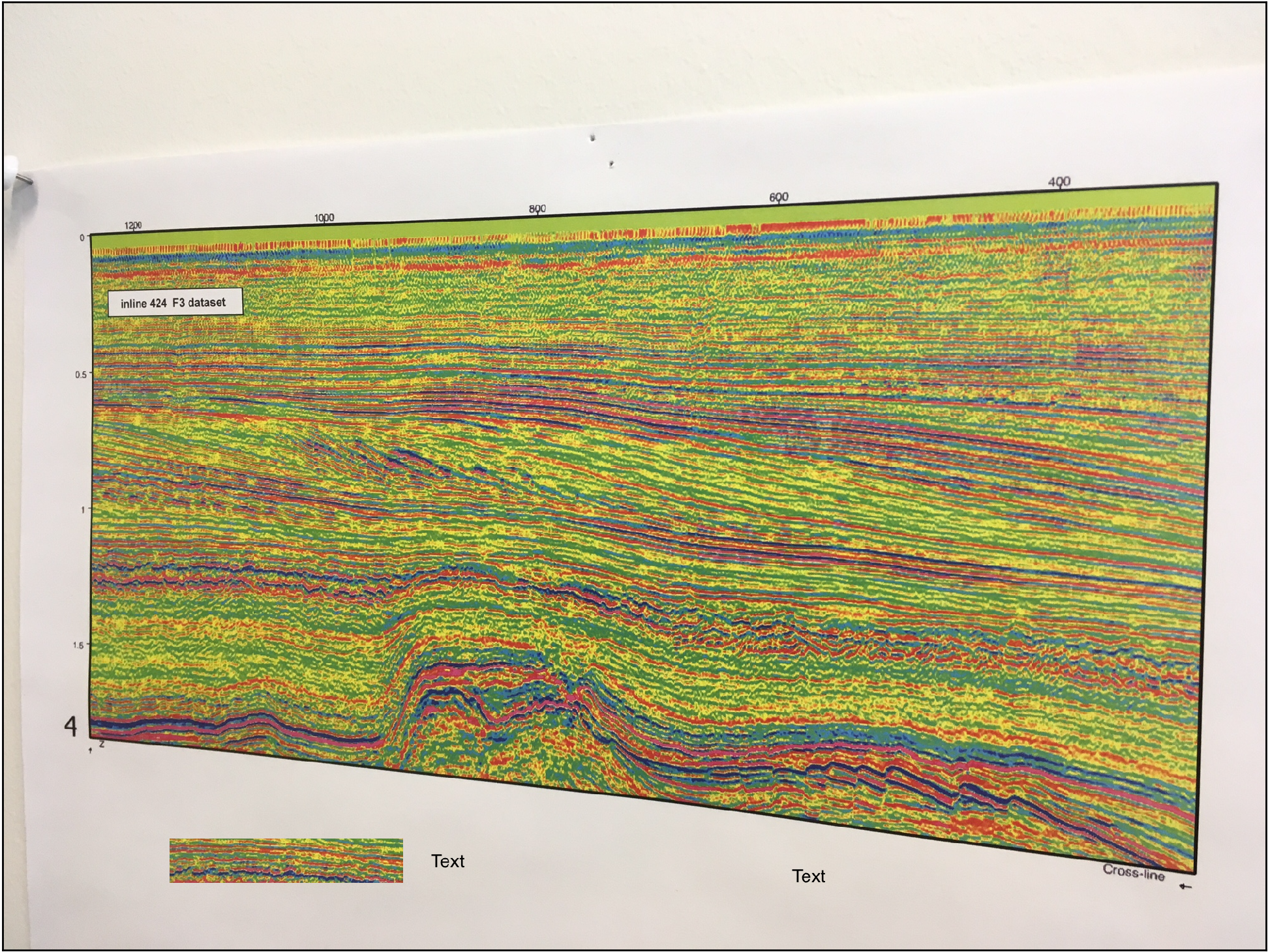Figure 1

The result was a single binary image with the white object representing the pixels occupied by the seismic section (Figure 2).Figure 2

You can download from GitHub all the tools for the automated workflow (including both part 1 and part 2, and some of the optional features outlined in the introduction) in the module mycarta.py, as well as an example Jupyter Notebook showing how to run it.

Next I want to use this binary object to derive a transformation function to rectify to a rectangle the seismic section in the input image.

The first step is to detect the contour of the object. Notice that because we used morphological operations it is not a perfect quadrilateral: it has rounded corners and some of the sides are bent, therefore the second step will be to approximate the contour with a polygon with enough tolerance to ensure it has 4 sides only(this took some trial and error but 25 turned out to be a good value for the parameter for a whole lot of test images I tried).

In reality, the two steps are performed together using the functions find_contours (there is only one to find, reallyand approximate_polygon from the skimage.measure module, as below:

```contour = np.squeeze(find_contours(enhanced, 0))
coords = approximate_polygon(contour, tolerance=25)```

The variable coords contains the coordinate for the corner points of the polygon (the first point is repeated last to close the polygon), which in Figure 3 I plotted superimposed to the input binary object.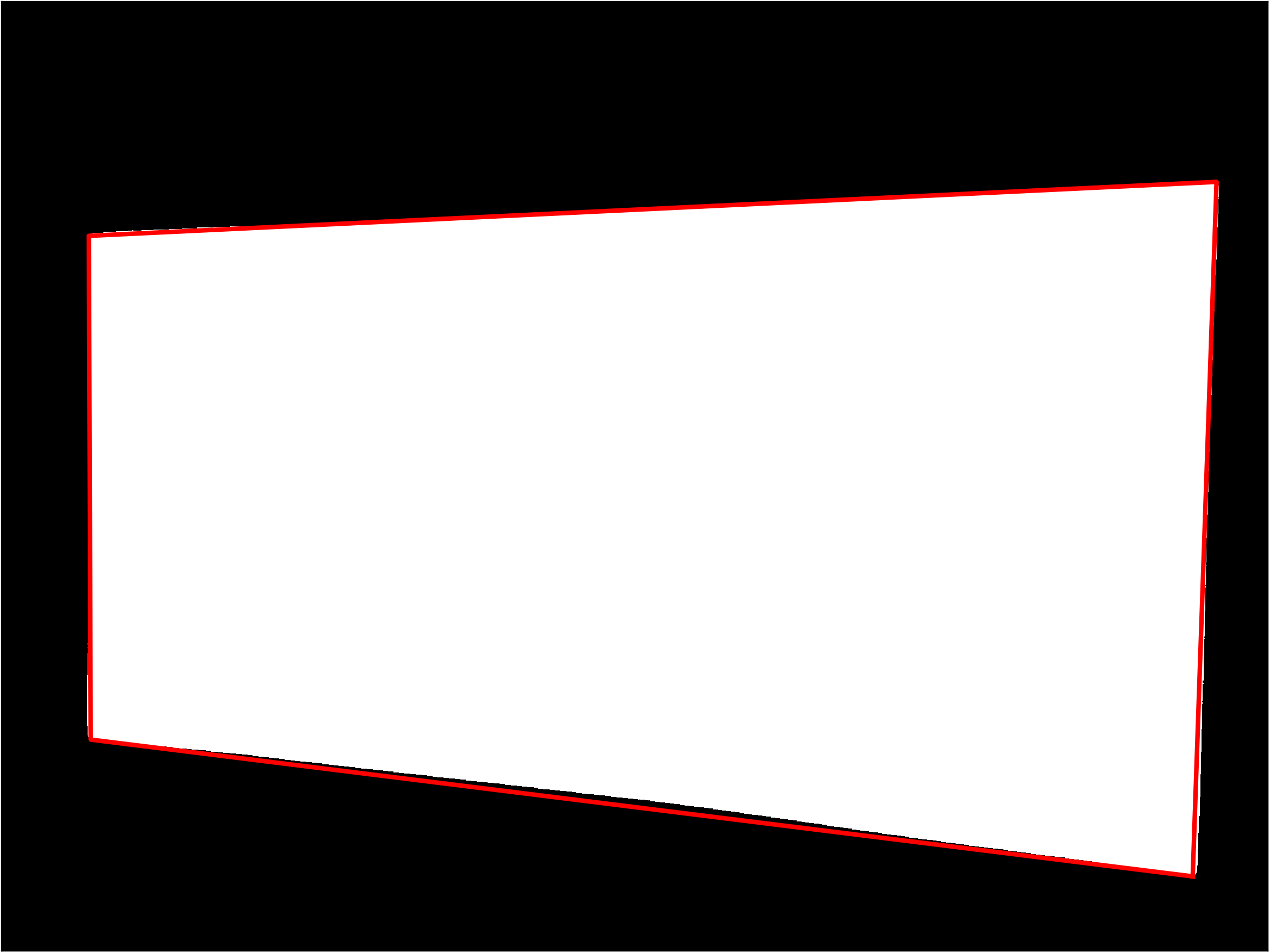Figure 3 – approximated polygon

A problem with the output of  approximate_polygon is that the points are not ordered; to solve it I adapted a function from a Stack Overflow answer to sort them based on the angle from their centroid:

```def ordered(points):
x = points[:,0]
y = points[:,1]
cx = np.mean(x)
cy = np.mean(y)
a = np.arctan2(y - cy, x - cx)
order = a.ravel().argsort()
x = x[order]
y = y[order]
return np.vstack([x,y])```

I call the function as below to get the corners in the contour without the last one (repetition of the first point).

`sortedCoords = ordered(coords[:-1]).T`

I can then plot them using colors in a predefined order to convince myself the indeed are sorted:

```plt.scatter(sortedCoords[:, 1], sortedCoords[:, 0], s=60,
color=['magenta', 'cyan', 'orange', 'green'])```Figure 4 – corners sorted in counter-clockwise order

The next bit of code may seem a bit complicated but it is not. With coordinates of the corners known, and their order as well, I can calculate the largest width and height of the input seismic section, and I use them to define the size of the registered output section, which is to be of rectangular shape:

```w1 = np.sqrt(((sortedCoords[0, 1]-sortedCoords[3, 1])**2)
+((sortedCoords[0, 0]-sortedCoords[3, 0])**2))
w2 = np.sqrt(((sortedCoords[1, 1]-sortedCoords[2, 1])**2)
+((sortedCoords[1, 0]-sortedCoords[2, 0])**2))

h1 = np.sqrt(((sortedCoords[0, 1]-sortedCoords[1, 1])**2)
+((sortedCoords[0, 0]-sortedCoords[1, 0])**2))
h2 = np.sqrt(((sortedCoords[3, 1]-sortedCoords[2, 1])**2)
+((sortedCoords[3, 0]-sortedCoords[2, 0])**2))

w = max(int(w1), int(w2))
h = max(int(h1), int(h2))```

and with those I define the coordinates of the output corners used to derive the transformation function:

```dst = np.array([
[0, 0],
[h-1, 0],
[h-1, w-1],
[0, w-1]], dtype = 'float32')```

Now I have everything I need to rectify the seismic section in the input image: it is warped using homologous points (the to sets of four corners) and a transformation function.

```dst[:,[0,1]] = dst[:,[1,0]]
sortedCoords[:,[0,1]] = sortedCoords[:,[1,0]]
tform = skimage.transform.ProjectiveTransform()
tform.estimate(dst,sortedCoords)
warped =skimage.transform.warp(img, tform, output_shape=(h-1, w-1))```

Notice that I had to swap the x and y coordinates to calculate the transformation function. The result is shown in Figure 5: et voilà!Figure 5 – rectified seismic section

You can download from GitHub the code to try this yourself (both part 1 and part 2, and some of the optional features outlined in the introduction, like removing the rectangle with label inside the section) as well as an example Jupyter Notebook showing how to run it.

# Computer vision in geoscience: recover seismic data from images – part 1

As anticipated in the introductory post of this short series I am going to demonstrate how to automatically detect where a seismic section is located in an image (be it a picture taken from your wall, or a screen capture from a research paper), rectify any distortions that might be present, and remove all sorts of annotations and trivia around and inside the section.

You can download from GitHub all the tools for the automated workflow (including both part 1 and part 2, and some of the optional features outlined in the introduction) in the module mycarta.py, as well as an example Jupyter Notebook showing how to run it.

In this part one I will be focusing on the image preparation and enhancement, and the automatic detection of the seismic section (all done using functions from numpy, scipy, and scikit-image)In order to do that, first I convert the input image  (Figure 1) containing the seismic section to grayscale and then enhance it by increasing the image contrast (Figure 2).Figure 1 – input image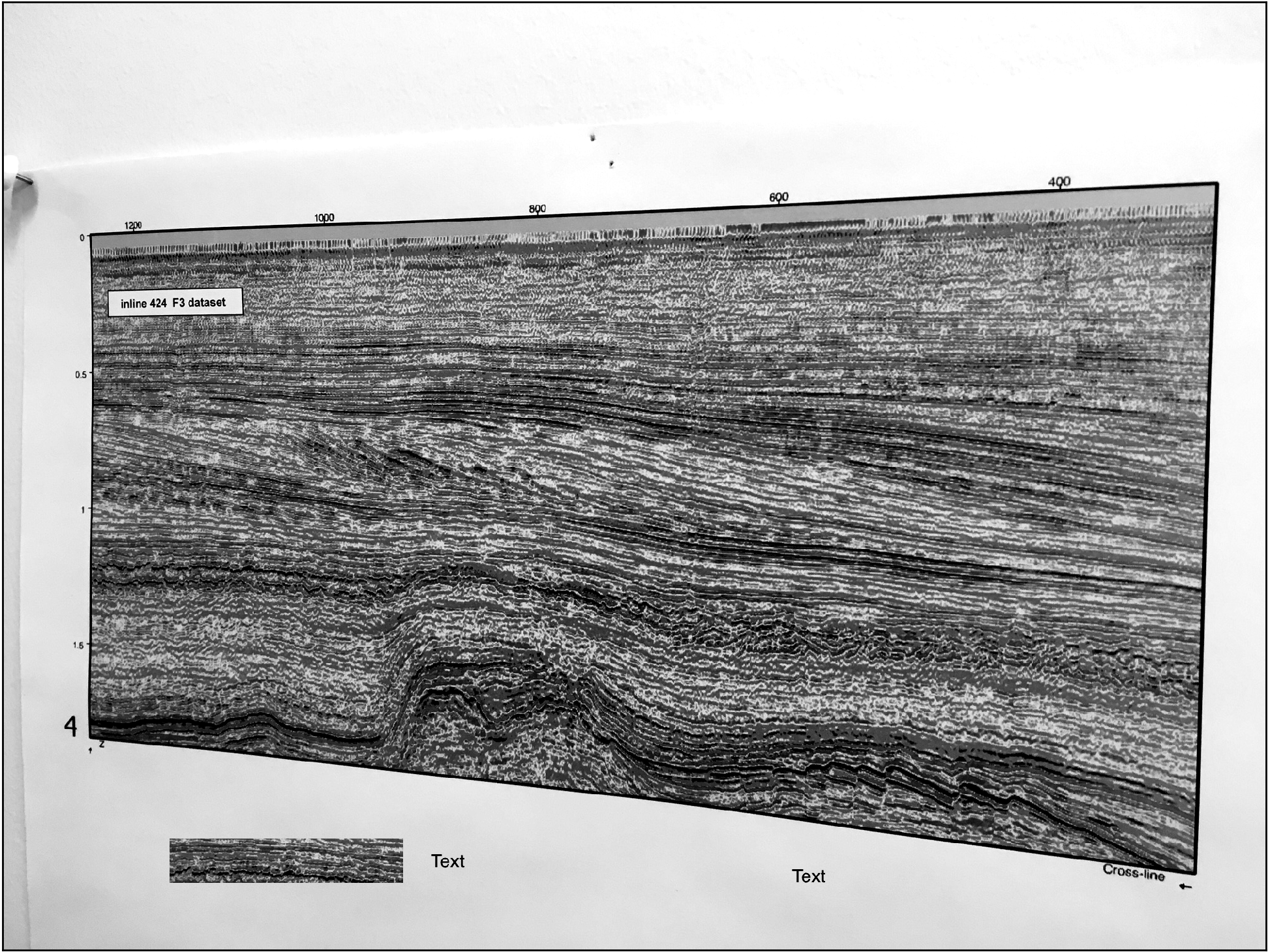Figure 2 – grayscale image

All it takes to do that is three lines of code as follows:

```gry = skimage.color.rgb2gray(img);
p2, p95 = numpy.percentile(gry, (2, 95))
rescale = exposure.rescale_intensity(gry, in_range=(p2, p95))```

For a good visual intuition of what actually is happening during the contrast stretching, check my post sketch2model – sketch image enhancements: in there  I show intensity profiles taken across the same image before and after the process.

Finding the seismic section in this image involve four steps:

1. converting the grayscale image to binary with a threshold (in this example a global threshold with the Otsu method)
2. finding and retaining only the largest object in the binary image (heuristically assumed to be the seismic section)
3. filling its holes
4. applying morphological operations to remove minutiae (tick marks and labels)

Below I list the code, and show the results.

```global_thresh = threshold_otsu(rescale)
binary_global = rescale < global_thresh```Figure 3 – binary image

```# (i) label all white objects (the ones in the binary image).
# scipy.ndimage.label actually labels 0s (the background) as 0 and then
# every non-connected, nonzero object as 1, 2, ... n.
label_objects, nb_labels = scipy.ndimage.label(binary_global)

# (ii) calculate every labeled object's binary size (including that
# of the background)
sizes = numpyp.bincount(label_objects.ravel())

# (3) set the size of the background to 0 so that if it happened to be
# larger than the largest white object it would not matter
sizes = 0

# (4) keep only the largest object
binary_objects = remove_small_objects(binary_global, max(sizes))

```Figure 4 – isolated seismic section

```# Remove holes (black regions inside white object)
binary_holes = scipy.ndimage.morphology.binary_fill_holes(binary_objects)```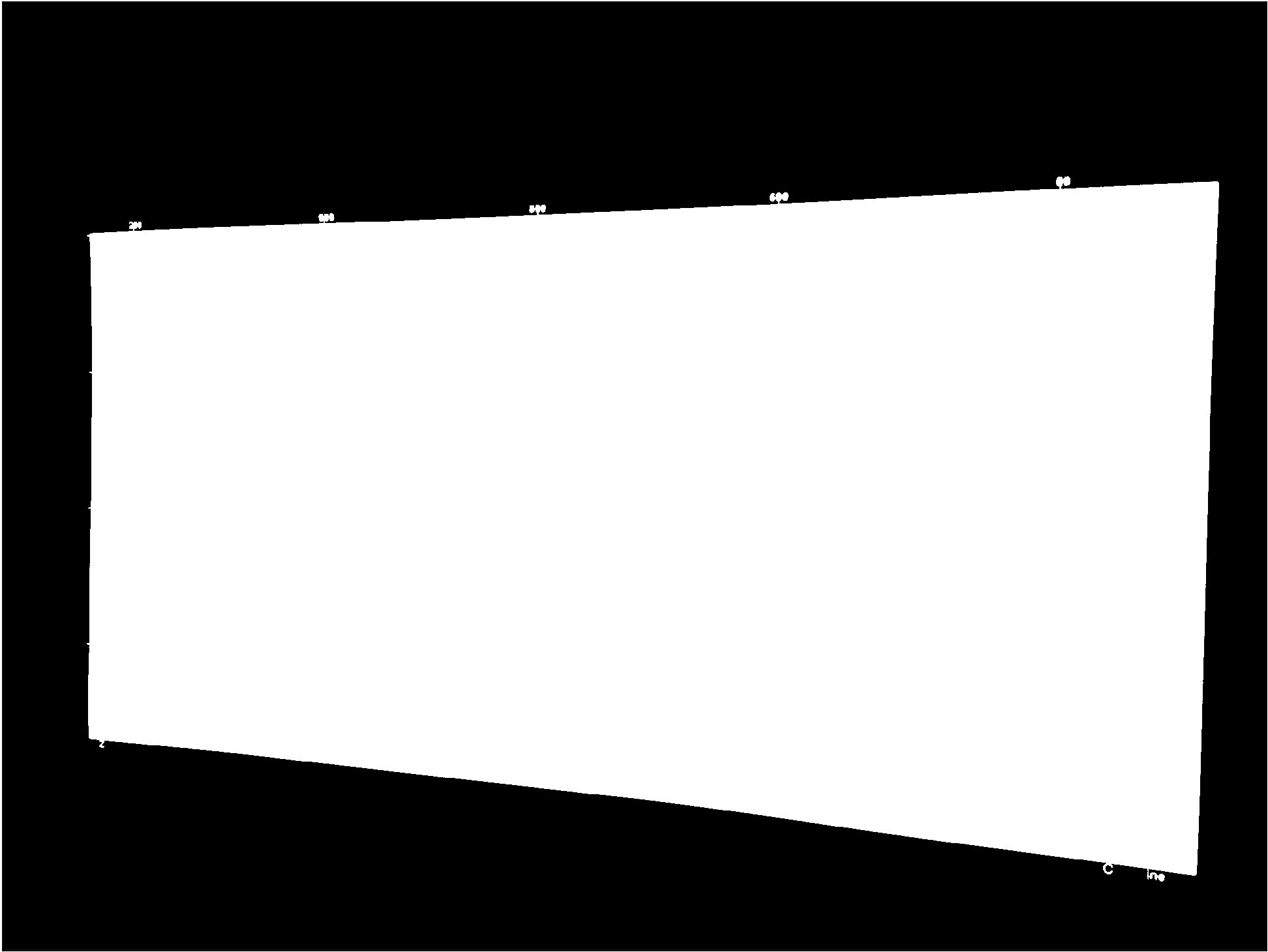Figure 5 – holes removed

`enhanced = opening(binary_holes, disk(7))`Figure 6 – removed residual tick marks and labels

That’s it!!!

You can download from GitHub all the tools for the automated workflow (including both part 1 and part 2, and some of the optional features outlined in the introduction) in the module mycarta.py, as well as an example Jupyter Notebook showing how to run it.

In the next post, we will use this polygonal binary object both as a basis to capture the actual coloured seismic section from the input image and to derive a transformation to rectify it to a rectangle.